## Doubled Tuned Coupled Circuits:

Figure 10.24 shows a Doubled Tuned Coupled Circuits involving two series resonant circuits.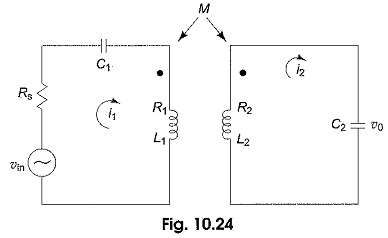For the circuit shown in the figure, a special case where the primary and secondary resonate at the same frequency ωr, is considered here,

i.e.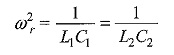The two mesh equations for the circuit are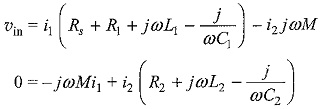From which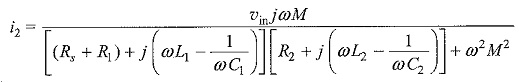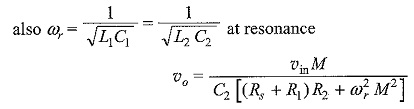or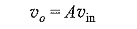where A is the amplification factor given by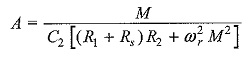The maximum amplification or the maximum output voltage can be obtained by taking the first derivative of υo with respect to M, and equating it to zero.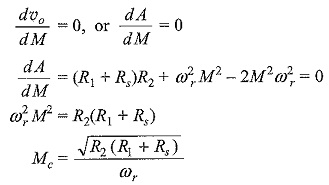where Mc is the critical value of mutual inductance. Substituting the value of Mc in the equation of υo we obtain the maximum output voltage as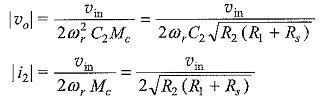By definition, M = K√L1L2, the coefficient of coupling, K at M = Mc is called the critical coefficient of coupling, and is given by Kc = Mc/√L2L1.The critical coupling causes the secondary current to have the maximum possible value. At resonance, the maximum value of amplification is obtained by changing M; or by changing the coupling coefficient for a given value of L1 and L2. The variation of output voltage with frequency for different coupling coefficients in Doubled Tuned Coupled Circuits is shown in Fig. 10.25.

Scroll to Top